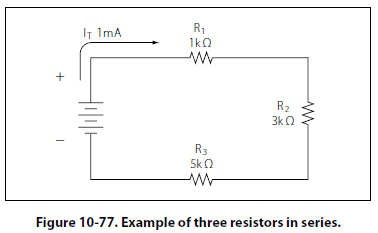Voltage Drops and Further Application of Ohm’s Law The example circuit in Figure 10-77 will be used to illustrate the idea of voltage drop. It is important to differentiate between voltage and voltage drop when discussing series circuits. Voltage drop refers to the loss in electrical pressure or emf caused by forcing electrons through a resistor. Because there are two resistors in the example, there will be separate voltage drops. Each drop is associated with each individual resistor. The amount of electrical pressure required to force a given number of electrons through a resistance is proportional to the size of the resistor.In Figure 10-77, the values used to illustrate the idea of voltage drop are: Current, I = 1mA R1 = 1 k O R2 = 3 k O R3 = 5 k O The voltage drop across each resistor will be calculated using Ohm’s law. The drop for each resistor is the product of each resistance and the total current in the circuit. Keep in mind that the same current flows through series resistor. Formula, E = I (R) Voltage across R1: E1 = IT (R1) E1 = 1 mA (1 k O) = 1 volt Voltage across R2: E2 = IT (R2) E2 = 1 mA (3 k O) = 3 volt Voltage across R3: E3 = IT (R3) E3 = 1 mA (5 k O) = 5 volt The source voltage can now be determined, which can then be used to confirm the calculations for each voltage drop. Using Ohm’s law: Formula: E = I (R) Source voltage = current times the total resistance ES = I (RT) RT = 1 k O + 3 k O + 5 k O RT = 9 k O Now: ES = I (RT) Substitute ES = 1 mA (9 k O) ES = 9 volts Simple checks to confirm the calculation and to illustrate the concept of the voltage drop add up the individual values of the voltage drops and compare them to the results of the above calculation. 1 volt + 3 volts + 5 volts = 9 volts.
 ©AvStop Online Magazine                                                                                                                                                      Contact Us              Return To Books